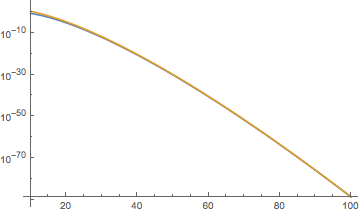# Asymptotics of Bessel functions

With $J_n$ standing for the Bessel function of first kind, $n\in \mathbb N$, I define $$f_n (\rho) =\int_0^π J_n(\rho \sin \theta) \sin \theta \ d\theta.$$ Assuming $1\ll\rho\ll n$, I would like to find an equivalent of $f_n(\rho)$. Is it a straightforward consequence of the known expansions?

• $f_n(\rho) = \pi J_{\frac{n-1}{2}}(\rho/2) J_{\frac{n+1}{2}}(\rho/2)$ may help. – Robert Israel Apr 7 '17 at 22:05

We could just take the large-$n$ asymptotic of $J_n(z)\rightarrow (2\pi n)^{-1/2}(ez/2n)^n$, and then

$$f_n(\rho)\rightarrow \frac{1}{n}(\tfrac{1}{2}e\rho/n)^n.$$

This seems to be quite reasonable in the desired range $1\ll\rho\ll n$:blue is the exact result, gold is the asymptotic expression, plotted as a function of $n$ for fixed $\rho=10$.

Large-n asymptotic expansions of the Bessel functions themselves have been derived by Olver and Temme. See http://dlmf.nist.gov/10.20 and http://dlmf.nist.gov/10.19 for formulas and citations. For practical purposes, they are very complicated and onerous to derive and evaluate, and they don't converge very quickly unless n is in the tens of thousands or above.

Given Robert Israel's result, the large-n expansion for $f_n(\rho)$ is just going to be an order-by-order multiplication of terms from the large-n Bessel expansions, and unless a dramatic simplification occurs, they are going to be complicated and onerous, too. I suggest you do the first two terms and see.